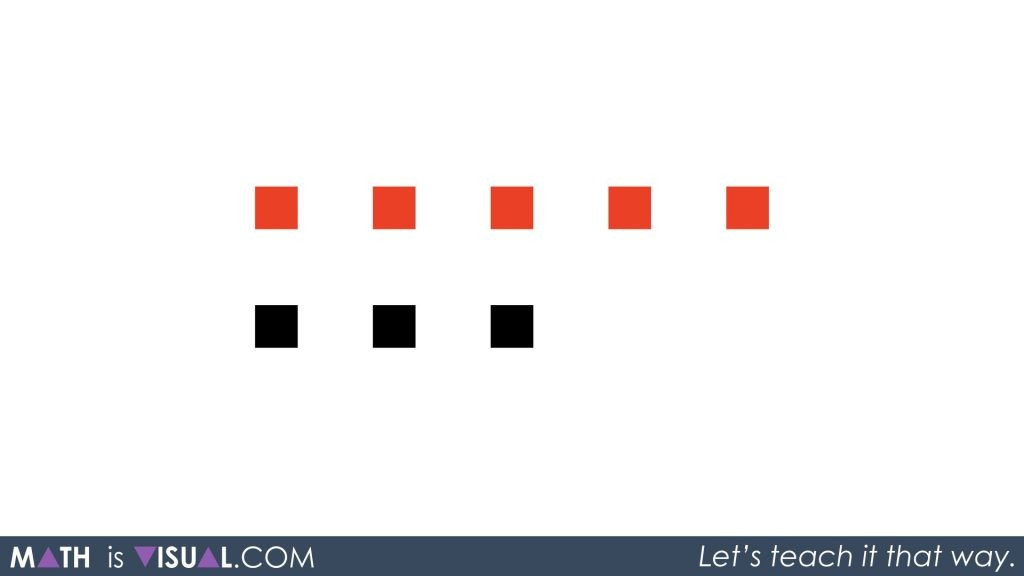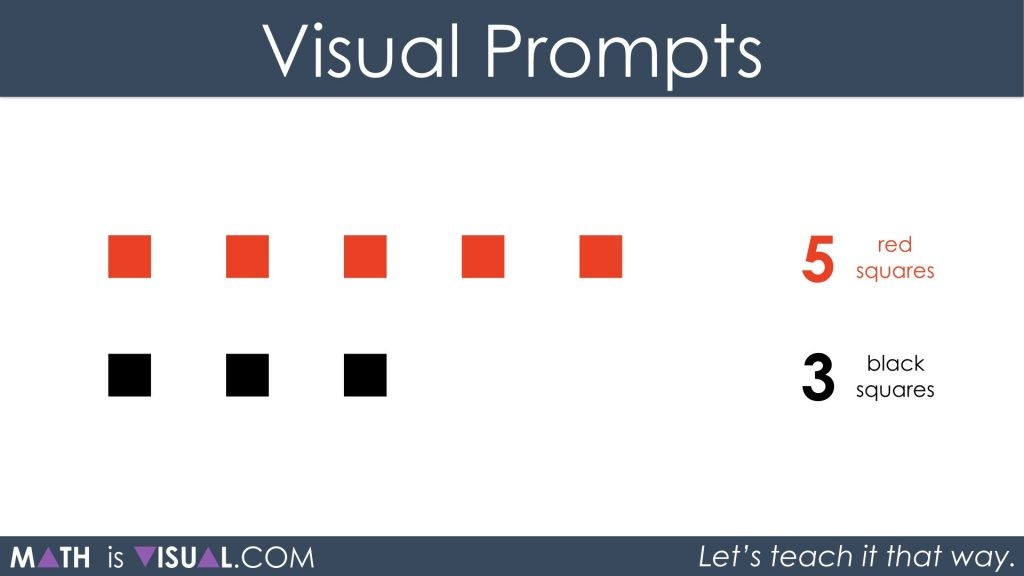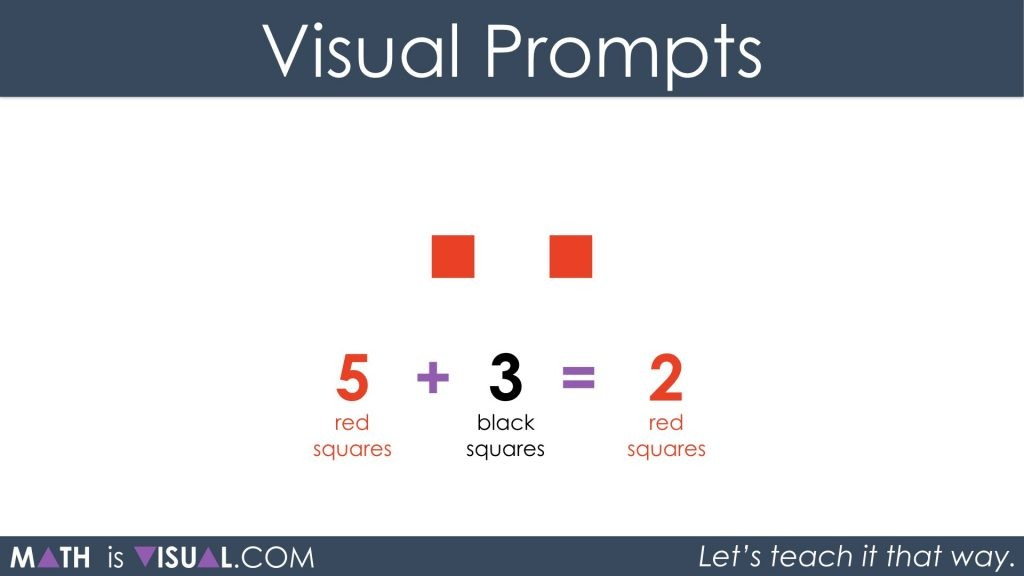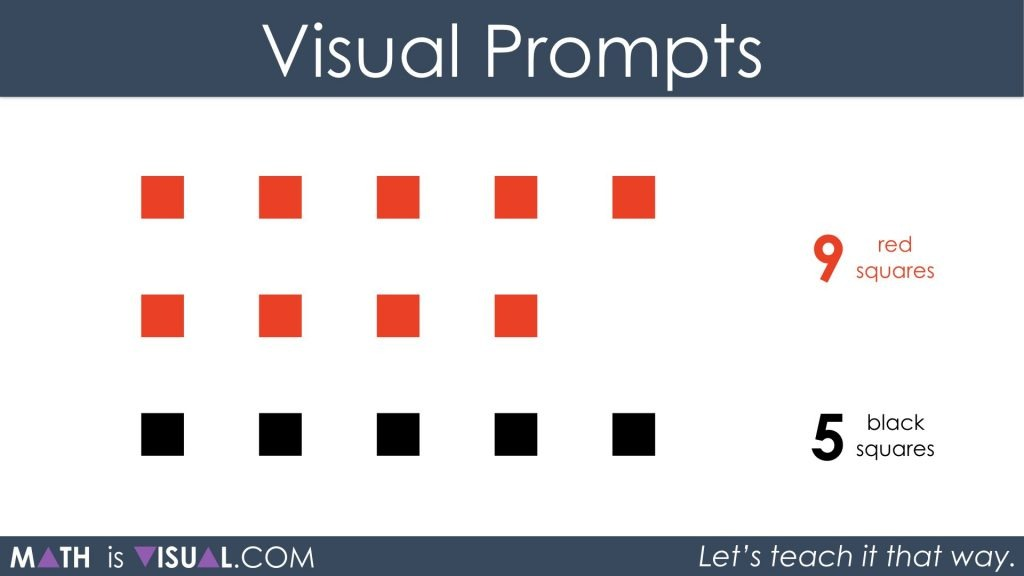# Adding Positive and Negative Integers, Part 2

Last time, we added positive and negative integers using black and red squares. Now we will show the red squares first and students see that the rules are the same.

In my previous post, we looked at adding and subtracting integers by implicitly introducing the idea of the Zero Principle through the use of squares on the screen. While some might interpret the black squares to represent positive values and red to represent negative, the choice is really up to the viewer.

This post introduces the red dots first. Under the assumption that many will believe the red to be negative, this post is intended to help students realize that order doesn’t matter; positive or negative.

Math is visual. Let’s teach it that way!

Use the following visual prompts to help students to conceptualize more difficult integer operation ideas like subtracting negative values (coming in the next couple posts).

## Math Is Visual Prompts

Feel free to use the video and pause in-between ideas or alternatively, use the following visual prompts.

#### Visual #1 Prompts

Show the following image:You may consider working only with the visual prior to introducing the numerical symbols included in the visual below:After giving students some time to determine how many squares will remain and what colour they will be, you can show them the visual that matches this scenario.#### Visual #2 Prompts

Show the following image:After students use square tiles and come to their conclusion, have them write out their thinking using a numerical expression.

Then, show them this:And finally, a simplified version: